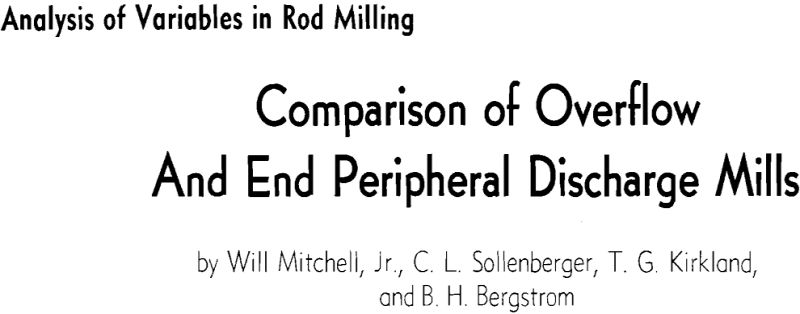# Comparison of Overflow and End Peripheral Discharge Mills

In a previous article the authors outlined a study of the variables in rod milling and also reported data from a series of open circuit grinding tests on a massive limestone in a 30-in. x 4-ft end peripheral discharge rod mill. As a second part of the experimental program, an analysis is now presented for the 30-in. x 4-ft overflow rod mill grinding under identical conditions, except that discharge ports on the periphery of the mill shell have been sealed so that the products from the present series overflowed through a 9-in. diam opening in the center of the end plate. A variance analysis has been made of the combined data for the two experiments, and performances of the two mills are compared here.

To test the consistency of the reporting of the sieved products, an averaged sieve analysis was calculated from the wet-dry plots obtained from the three product samples of each run. The resulting averaged analysis was plotted and the P80 selected. The relative deviations of the P80’s from each of the three product samples with respect to the P80 of the averaged analysis were then calculated. In only two sets were the relative deviations (6.2 and 9.9 pct) considered excessive. In each of these two sets, one sieve analysis was obviously out of line; hence that analysis was ignored and new averages were computed. This reduced the relative deviations to 1.2 and 2.7 pct respectively.

Only the adjusted work index values, not the actual experimental values, were used for the variance analyses and for the graphs.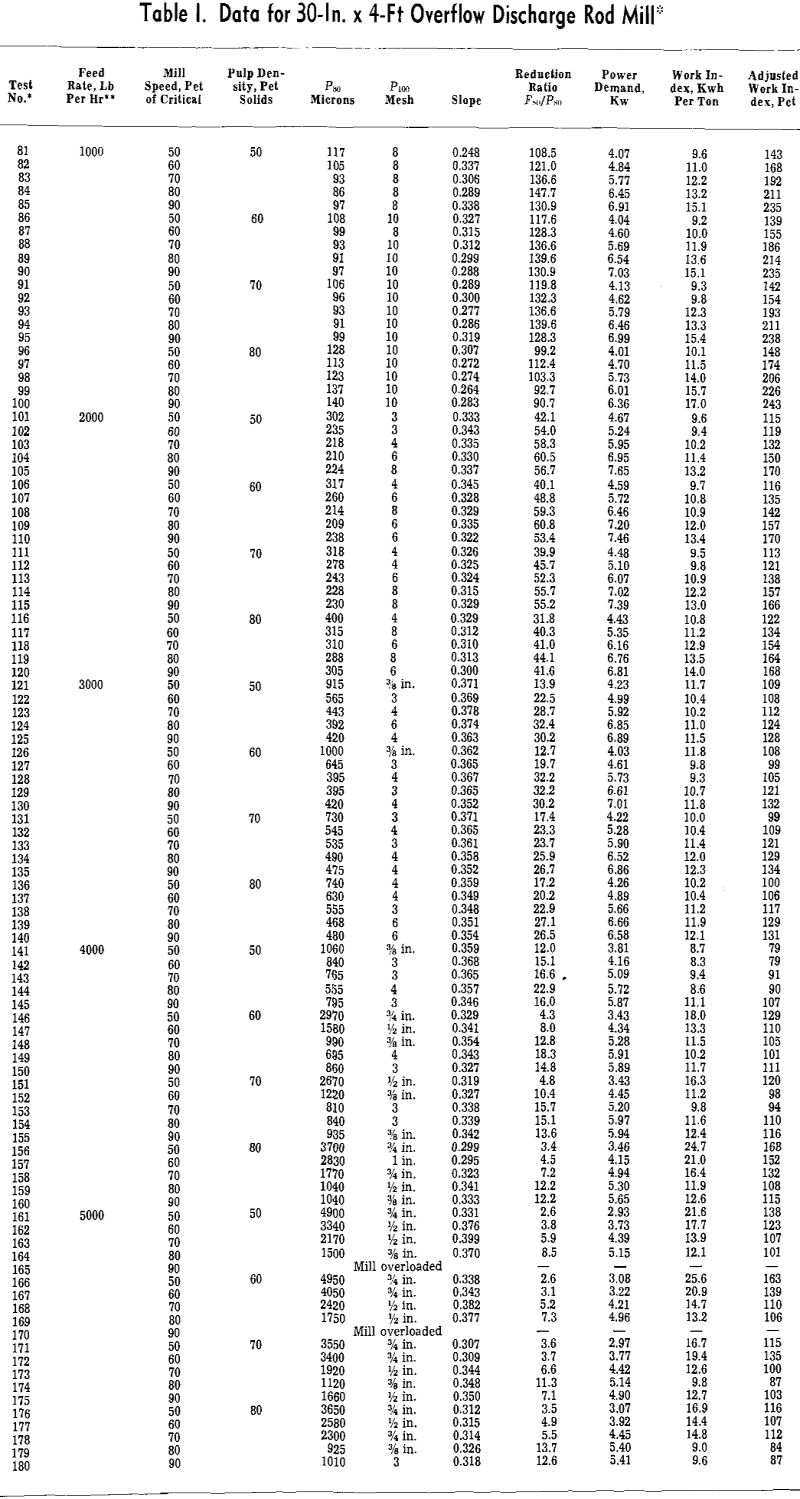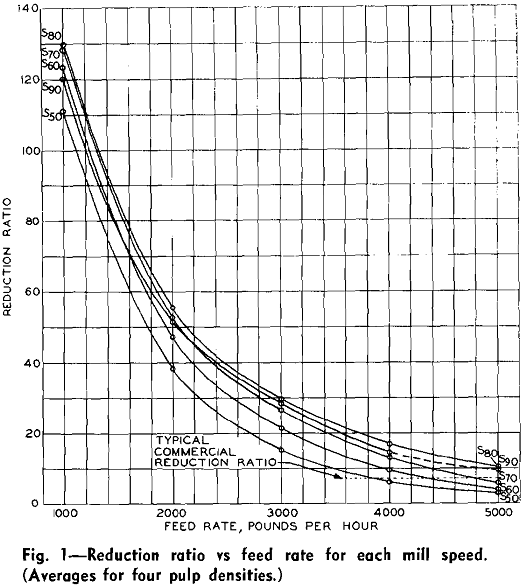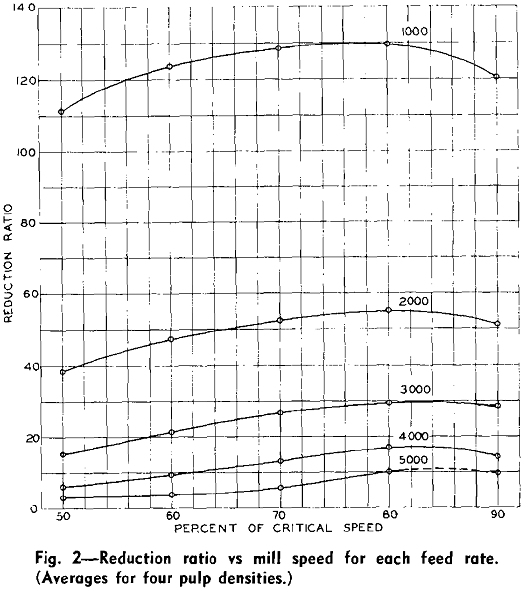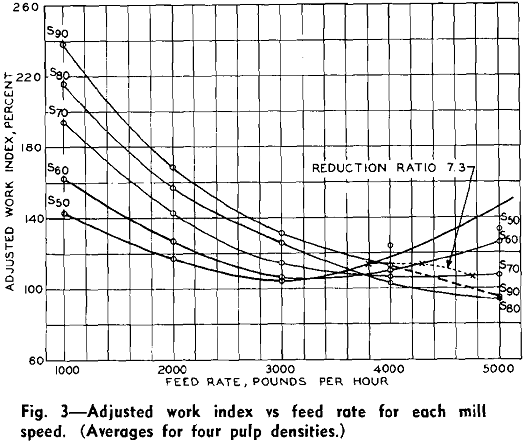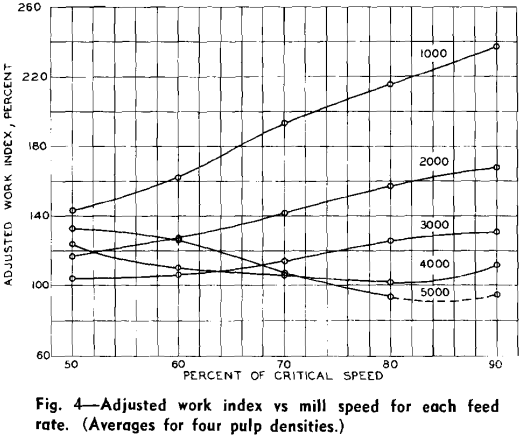Reduction Ratio: Feed rate, mill speed, and an interaction of feed rate and pulp density were found to have a significant effect upon reduction ratio, all at the 0.1 pct or the highest level of significance. Because pulp density was not shown to be significant as a main effect, it was averaged out of all of the data.

To determine the reason for the feed rate—pulp density interaction, the data were examined without the pulp density being averaged out. Pulp density appeared to have little effect on reduction ratio at feed rates of 3000, 4000, and 5000 lb per hr. At 1000 and 2000 lb per hr, however, lower reduction ratios were obtained at 80 pct solids than were obtained at the lower pulp densities.

Adjusted Work Index: Although no test was available for the significance of feed rate it appeared to have a substantial effect on work index. Mill speed was significant at the 5 pct or lowest level, and interactions of feed rate with mill speed and pulp density were significant at the 0.1 pct level.

Separate variance analyses, at each feed rate, of the data including the pulp density variable revealed that pulp density was not significant at 3000 or 5000 lb per hr but was significant at all other feed rates. This explained the feed rate-pulp density interaction. An examination of the data indicated that at the feed rates where pulp density was significant, higher grinding efficiencies were obtained at 50 to 70 pct solids than at 80 pct solids

Reduction Ratio: The variance analysis revealed that reduction ratio was significantly affected by feed rate (5 pct level) and mill speed (0.1 pct level) when both mills were considered. Type of discharge, however, was not significant as a main effect, but only as an interaction with feed rate (0.1 pct level).

At a constant reduction ratio of 7.3, a 10 to 15 pct increase in capacity was noted for both mills as mill speed was increased from 50 to 60 and from 60 to 70 pct of critical. This increase in capacity was obtained in both cases at slightly greater efficiencies.